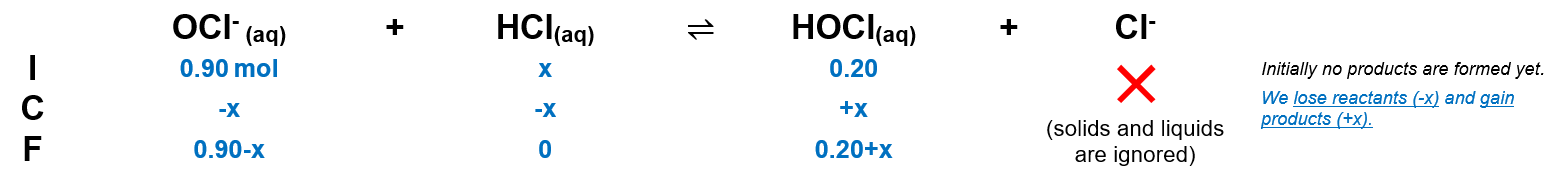# Problem: Calculate the pH of a solution that is 0.20 M HOCl and 0.90 M KOCl. In order for this buffer to have pH = pKa, would you add HCl or NaOH? What quantity (moles) of which reagent would you add to 1.0 L of the original buffer so that the resulting solution has pH = pKa?

###### FREE Expert Solution

We are asked to calculate the pH of a solution that is 0.20 M HOCl and 0.90 M KOCl.

Use buffer equation:

Given:

pKa = 7.53 (from standard tables)

[A-] →  KOCl

[HA] → HOCl

Calculate pH:

pH = 8.18

The pH of a solution that is 0.20 M HOCl and 0.90 M KOCl is pH = 8.18

The ratio [A-]/[HA] = 1 so that pKa = pH.

• Our Initial [A-]/[HA] = 4.5
• This means we need to remove the conjugate base and produce more acid to lessen the ratio.
• This means we need to add more HCl.

Calculate used HCl:94% (365 ratings)###### Problem Details

Calculate the pH of a solution that is 0.20 M HOCl and 0.90 M KOCl. In order for this buffer to have pH = pKa, would you add HCl or NaOH? What quantity (moles) of which reagent would you add to 1.0 L of the original buffer so that the resulting solution has pH = pKa?